# Impact of bit collisions on learning performance

In an earlier post I looked at the minimal impact bit collisions in the RDKit's Morgan fingerprints has on calculated similarity between molecules. This time I'm going to look at the impact on the performance of machine-learning algorithms.

I will use Datasets II from our model fusion paper to do the analysis. I've looked at these datasets, which are available as part of the benchmarkng platform in some detail in an earlier post.

The benchmarking platform is pre-configured to support a short (1K) and long (16K) form of the RDKit Morgan2 fingerprint, so I didn't need to make any changes to the platform itself for this analysis.

In :
```import cPickle,glob,gzip
import numpy as np
from __future__ import print_function

import time
print(time.asctime())
```
```Sun Mar 16 04:58:46 2014

```

Read in the results:

In :
```alld = {}
for fn in glob.glob('../../Code/benchmarking_platform/validation/data_sets_II/ChEMBL/*.pkl.gz'):
d = cPickle.load(gzip.open(fn))
fn = fn.split('_')[-1].split('.')
alld[fn]=d
```

We'll start with logisitic regression.

For each dataset, calculate the mean AUC, EF1, and EF5 for models built using the two different fingerprints. Also calculated the mean, min, and max delta between the the fingerprints.

In :
```means={}
delts={}
fdelts={}
for assay,d in alld.items():
v1 = np.array([x for x in d['AUC']['lr_ecfp4']])
v2 = np.array([x for x in d['AUC']['lr_lecfp4']])
delt=v2-v1
means[assay]={'AUC':(np.mean(v1),np.mean(v2))}
delts[assay]={'AUC':(np.mean(delt),np.min(delt),np.max(delt))}
fdelts[assay]={'AUC':delt}
v1 = np.array([x for x in d['EF1']['lr_ecfp4']])
v2 = np.array([x for x in d['EF1']['lr_lecfp4']])
delt=v2-v1
means[assay]['EF1']=(np.mean(v1),np.mean(v2))
delts[assay]['EF1']=(np.mean(delt),np.min(delt),np.max(delt))
fdelts[assay]['EF1']=delt
v1 = np.array([x for x in d['EF5']['lr_ecfp4']])
v2 = np.array([x for x in d['EF5']['lr_lecfp4']])
delt=v2-v1
means[assay]['EF5']=(np.mean(v1),np.mean(v2))
delts[assay]['EF5']=(np.mean(delt),np.min(delt),np.max(delt))
fdelts[assay]['EF5']=delt

```

Look at the mean values of the metrics across the different targets.

In :
```figsize(16,12)
subplot(3,1,1)
plot([x['AUC'] for x in means.values()],c='b')
plot([x['AUC'] for x in means.values()],c='r')
title('AUC')
subplot(3,1,2)
plot([x['EF5'] for x in means.values()],c='b')
plot([x['EF5'] for x in means.values()],c='r')
title('EF5')
subplot(3,1,3)
plot([x['EF1'] for x in means.values()],c='b')
plot([x['EF1'] for x in means.values()],c='r')
_=title('EF1')
```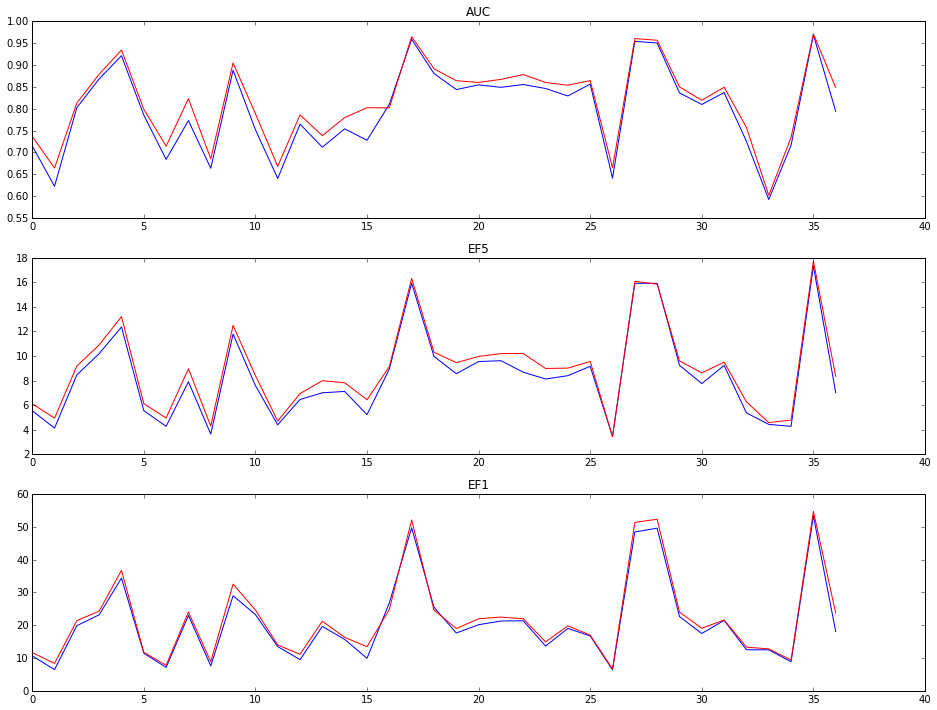With LR, at least, the longer fingerprints (red lines) are, on average, slightly better with each metric.

Using the means here, which are calculated across multiple datasets (papers) per target isn't the most accurate way to view the results, it's more accurate to look at the deltas.

The same behavior is observed in the deltas, though the min delta curves do show that there are times that the longer fingerprints are sligthly worse:

In :
```figsize(16,12)
subplot(3,1,1)
plot([x['AUC'] for x in delts.values()],c='b')
plot([x['AUC'] for x in delts.values()],c='r')
plot([x['AUC'] for x in delts.values()],c='r')
title(r'\$\Delta\$ AUC')
subplot(3,1,2)
plot([x['EF5'] for x in delts.values()],c='b')
plot([x['EF5'] for x in delts.values()],c='r')
plot([x['EF5'] for x in delts.values()],c='r')
title(r'\$\Delta\$ EF5')
subplot(3,1,3)
plot([x['EF1'] for x in delts.values()],c='b')
plot([x['EF1'] for x in delts.values()],c='r')
plot([x['EF1'] for x in delts.values()],c='r')
_=title(r'\$\Delta\$ EF1')
```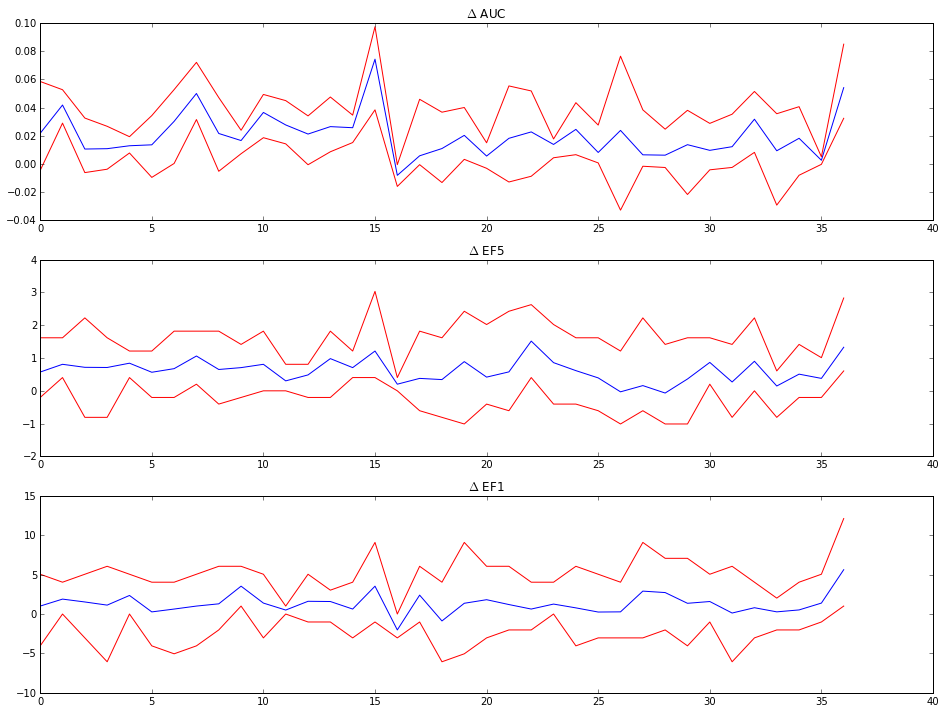That's a bit clearer in a box plot for the deltas:

In :
```figsize(16,12)
subplot(3,1,1)
_=boxplot([x['AUC'] for x in fdelts.values()])
_=plot((0,38),(0,0),c='k',linestyle='--')
title(r'\$\Delta\$ AUC')

subplot(3,1,2)
_=boxplot([x['EF5'] for x in fdelts.values()])
_=plot((0,38),(0,0),c='k',linestyle='--')
title(r'\$\Delta\$ EF5')

subplot(3,1,3)
_=boxplot([x['EF1'] for x in fdelts.values()])
_=plot((0,38),(0,0),c='k',linestyle='--')
_=title(r'\$\Delta\$ EF1')
```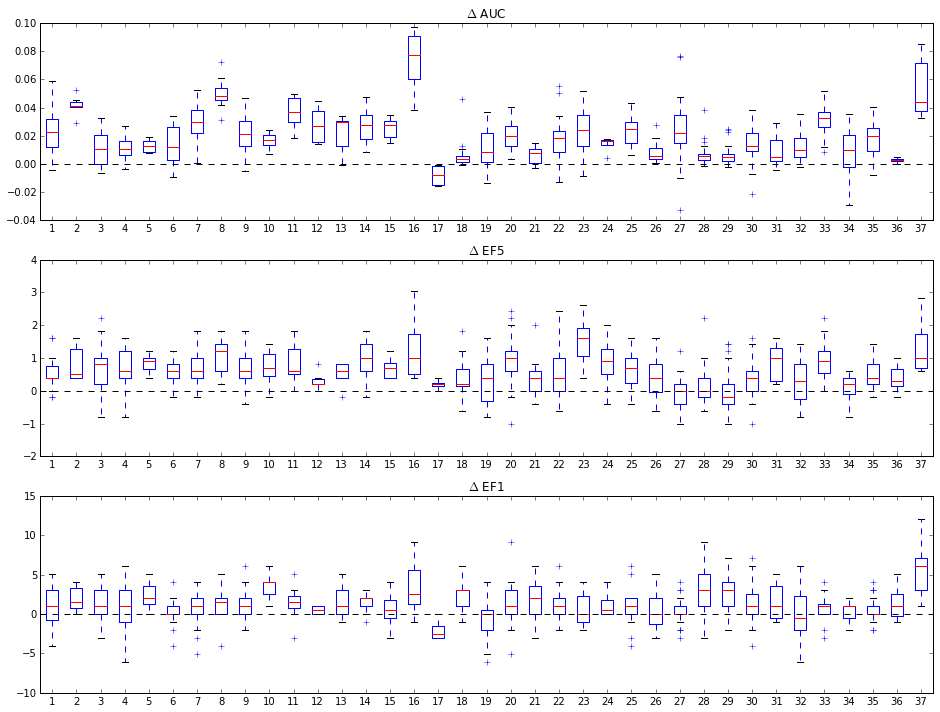Repeat for Naive Bayes

In :
```means={}
delts={}
fdelts={}
for assay,d in alld.items():
v1 = np.array([x for x in d['AUC']['nb_ecfp4']])
v2 = np.array([x for x in d['AUC']['nb_lecfp4']])
delt=v2-v1
means[assay]={'AUC':(np.mean(v1),np.mean(v2))}
delts[assay]={'AUC':(np.mean(delt),np.min(delt),np.max(delt))}
fdelts[assay]={'AUC':delt}
v1 = np.array([x for x in d['EF1']['nb_ecfp4']])
v2 = np.array([x for x in d['EF1']['nb_lecfp4']])
delt=v2-v1
means[assay]['EF1']=(np.mean(v1),np.mean(v2))
delts[assay]['EF1']=(np.mean(delt),np.min(delt),np.max(delt))
fdelts[assay]['EF1']=delt
v1 = np.array([x for x in d['EF5']['nb_ecfp4']])
v2 = np.array([x for x in d['EF5']['nb_lecfp4']])
delt=v2-v1
means[assay]['EF5']=(np.mean(v1),np.mean(v2))
delts[assay]['EF5']=(np.mean(delt),np.min(delt),np.max(delt))
fdelts[assay]['EF5']=delt

```
In :
```figsize(16,12)
subplot(3,1,1)
plot([x['AUC'] for x in means.values()],c='b')
plot([x['AUC'] for x in means.values()],c='r')
title('AUC')
subplot(3,1,2)
plot([x['EF5'] for x in means.values()],c='b')
plot([x['EF5'] for x in means.values()],c='r')
title('EF5')
subplot(3,1,3)
plot([x['EF1'] for x in means.values()],c='b')
plot([x['EF1'] for x in means.values()],c='r')
_=title('EF1')
```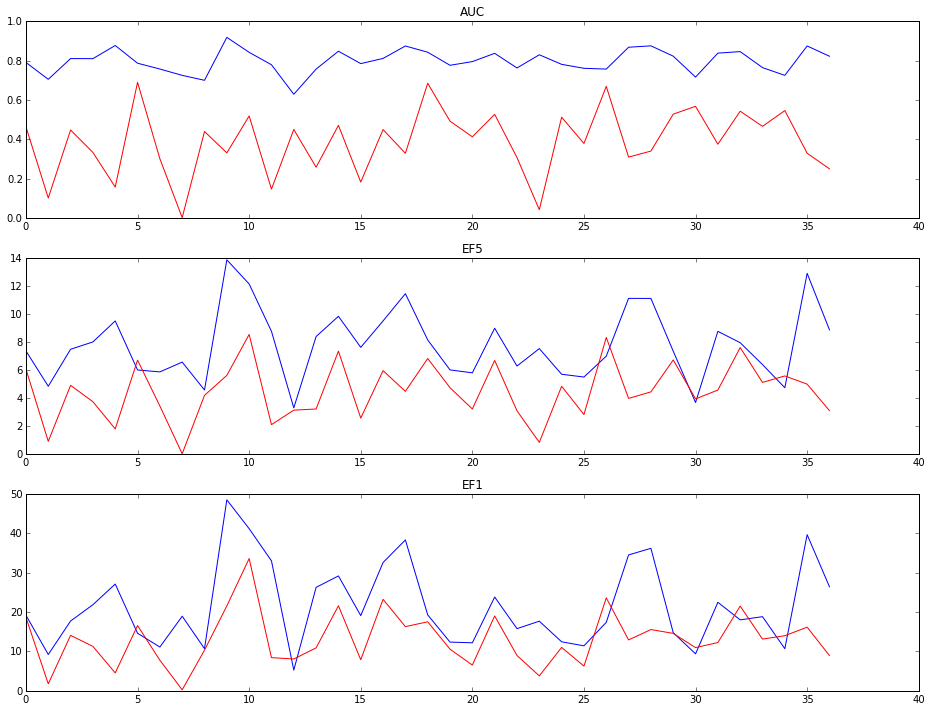In :
```figsize(16,12)
subplot(3,1,1)
_=boxplot([x['AUC'] for x in fdelts.values()])
_=plot((0,38),(0,0),c='k',linestyle='--')
title(r'\$\Delta\$ AUC')

subplot(3,1,2)
_=boxplot([x['EF5'] for x in fdelts.values()])
_=plot((0,38),(0,0),c='k',linestyle='--')
title(r'\$\Delta\$ EF5')

subplot(3,1,3)
_=boxplot([x['EF1'] for x in fdelts.values()])
_=plot((0,38),(0,0),c='k',linestyle='--')
_=title(r'\$\Delta\$ EF1')
```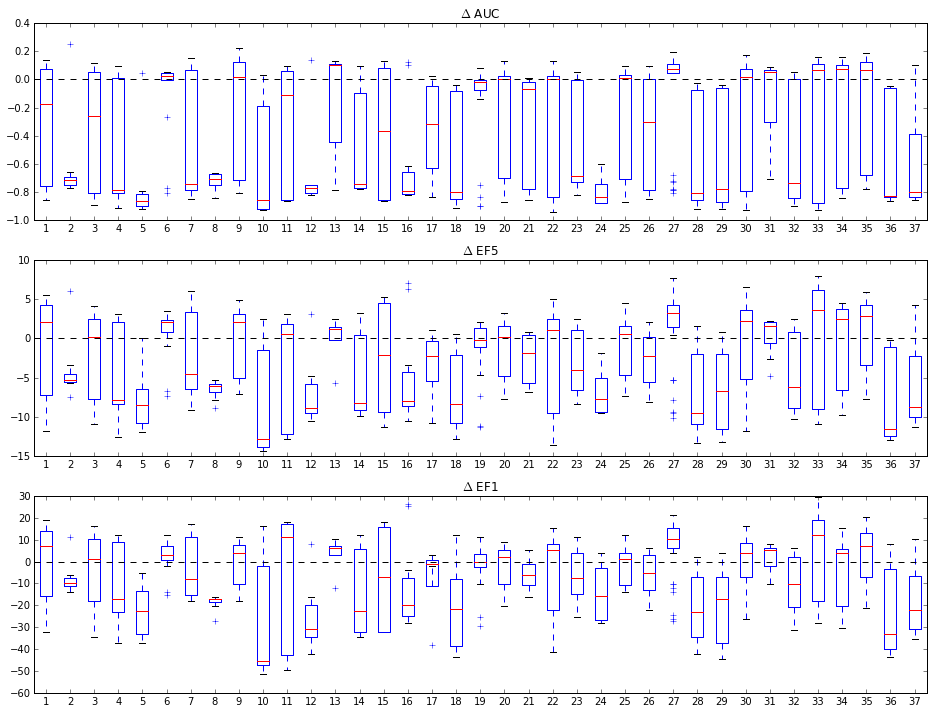Wow is that a mess. Removing the collisions (adding more set bits) dramatically drops the performance of the NB classifier. This matches something we saw while putting together the fusion paper: the performance of NB degrades in the face of a large number of low-signal bits.

Now move on to random forests

In :
```means={}
delts={}
fdelts={}
for assay,d in alld.items():
v1 = np.array([x for x in d['AUC']['rf_ecfp4']])
v2 = np.array([x for x in d['AUC']['rf_lecfp4']])
delt=v2-v1
means[assay]={'AUC':(np.mean(v1),np.mean(v2))}
delts[assay]={'AUC':(np.mean(delt),np.min(delt),np.max(delt))}
fdelts[assay]={'AUC':delt}
v1 = np.array([x for x in d['EF1']['rf_ecfp4']])
v2 = np.array([x for x in d['EF1']['rf_lecfp4']])
delt=v2-v1
means[assay]['EF1']=(np.mean(v1),np.mean(v2))
delts[assay]['EF1']=(np.mean(delt),np.min(delt),np.max(delt))
fdelts[assay]['EF1']=delt
v1 = np.array([x for x in d['EF5']['rf_ecfp4']])
v2 = np.array([x for x in d['EF5']['rf_lecfp4']])
delt=v2-v1
means[assay]['EF5']=(np.mean(v1),np.mean(v2))
delts[assay]['EF5']=(np.mean(delt),np.min(delt),np.max(delt))
fdelts[assay]['EF5']=delt

```
In :
```figsize(16,12)
subplot(3,1,1)
plot([x['AUC'] for x in means.values()],c='b')
plot([x['AUC'] for x in means.values()],c='r')
title('AUC')
subplot(3,1,2)
plot([x['EF5'] for x in means.values()],c='b')
plot([x['EF5'] for x in means.values()],c='r')
title('EF5')
subplot(3,1,3)
plot([x['EF1'] for x in means.values()],c='b')
plot([x['EF1'] for x in means.values()],c='r')
_=title('EF1')
```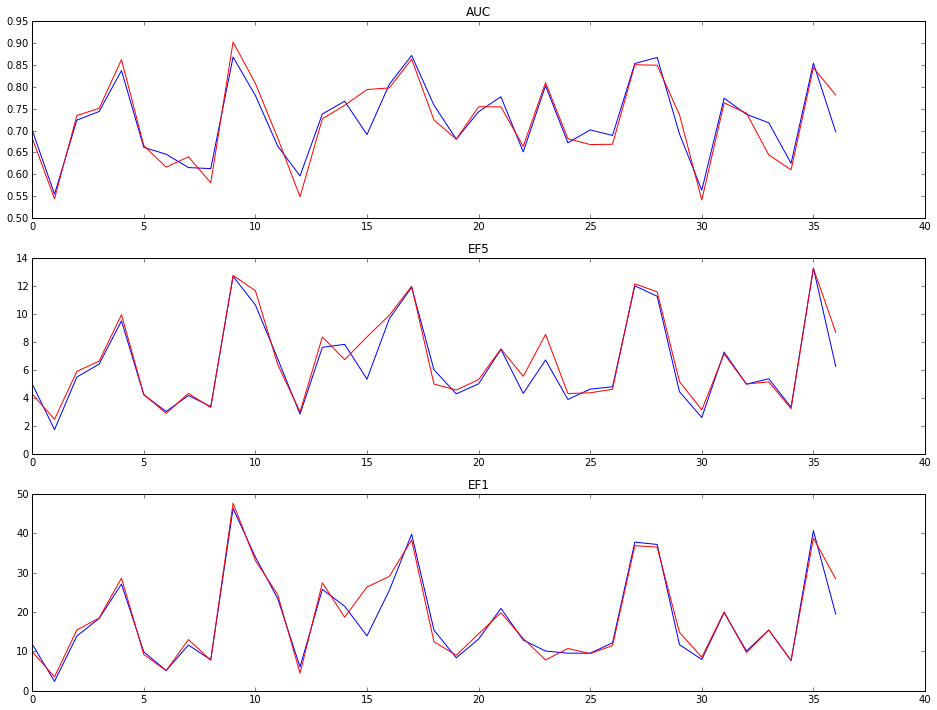In :
```figsize(16,12)
subplot(3,1,1)
_=boxplot([x['AUC'] for x in fdelts.values()])
_=plot((0,38),(0,0),c='k',linestyle='--')
title(r'\$\Delta\$ AUC')

subplot(3,1,2)
_=boxplot([x['EF5'] for x in fdelts.values()])
_=plot((0,38),(0,0),c='k',linestyle='--')
title(r'\$\Delta\$ EF5')

subplot(3,1,3)
_=boxplot([x['EF1'] for x in fdelts.values()])
_=plot((0,38),(0,0),c='k',linestyle='--')
_=title(r'\$\Delta\$ EF1')
```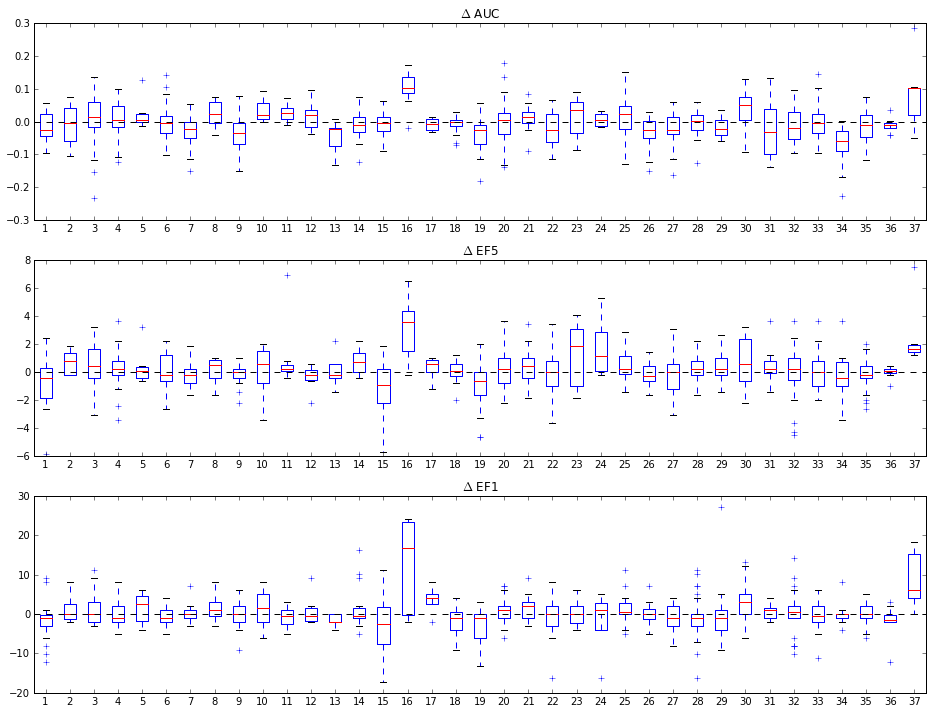It would be easy to reach the conclusion that the RF models are least sensitive to removing bit collisions. However, note that the y scales on these plots are larger than those for the LR models. Redoing the plots on the same y scale shows that there is considerable more variation within the datasets for each target and more examples where the additional resolution hurts the accuracy:

In :
```figsize(16,12)
subplot(3,1,1)
_=boxplot([x['AUC'] for x in fdelts.values()])
_=plot((0,38),(0,0),c='k',linestyle='--')
ylim(-.04,.1)
title(r'\$\Delta\$ AUC')

subplot(3,1,2)
_=boxplot([x['EF5'] for x in fdelts.values()])
_=plot((0,38),(0,0),c='k',linestyle='--')
ylim(-2,4)
title(r'\$\Delta\$ EF5')

subplot(3,1,3)
_=boxplot([x['EF1'] for x in fdelts.values()])
_=plot((0,38),(0,0),c='k',linestyle='--')
ylim(-10,15)
_=title(r'\$\Delta\$ EF1')
```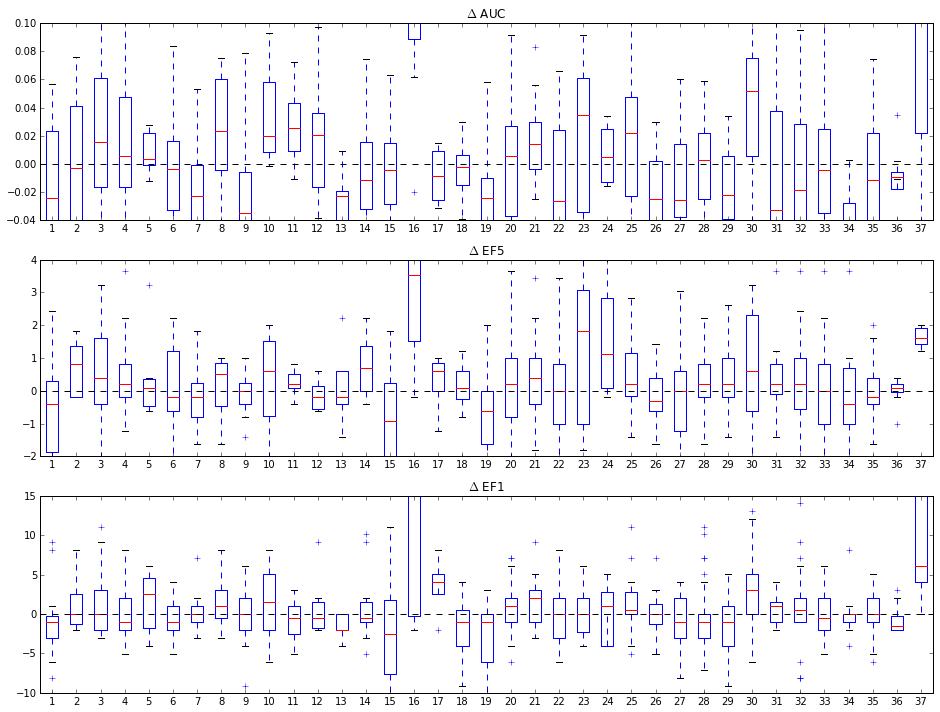In []: